Contenus

## Corrected exercises: Queue

The corrected exercises below concern the Markov chains in continuous time, and more particularly the notion of waiting line.

## Exercise 1

A client-server system receives an average of 1000 requests per second, arriving at a Poisson process. It has a single server that can process an average of 2000 clients per second. It is assumed that the service time of a customer is distributed according to the exponential law. Calculate the probability that the service time exceeds 2 ms.

What is the percentage of rejected customers for a system that does not have a queue.

Same question for a system with a 1-seat queue. Calculate the server application rate.

Same question for a system with a queue of 2 places.

By choosing the millisecond as the unit of time, we obtain a birth rate of 1 and a death rate of 2. The service time TS of a customer follows an exponential law of parameter 2 therefore the probability that the service time exceeds two seconds is 0.0183 thanks to the following formula: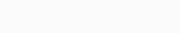Chain (a) represents a capacity in the 0 seat queue while chain (b) represents a capacity in the 1 seat queue. For each new place, it suffices to add a state.

For the first chain, we will calculate the stationary distribution:We therefore have 1/3 of rejected clients (if we are 1/3 of the time in state 1, then we can no longer receive clients on 1/3 of the functioning of the queue). The occupancy rate is pi0 = 2/3.

For the second chain, we will calculate the stationary distribution:And for a capacity of 2 in the queue we get pi3 = 1/15 and pi0 = 8/15.

## Exercise 2

A client-server system receives an average of 1000 requests per second, arriving in a Poisson process. As an experiment, we are considering a system without a queue but comprising several front-end servers. When all servers are busy, requests are rejected.

What is the percentage of rejected clients for a system with 1 server processing 4000 requests per second

Same question for a system with two servers each processing 2000 requests per second.

Same question for a system with four servers each processing 1000 requests per second.

Regardless of the number of servers, the idea of parallel computing without queuing is always constructed in the same way: with each new client, one more server operates.Just calculate the stationary distribution, the number of rejected clients is equal to the distribution of the last state (the most to the right here).

For a server: 1/5

For two servers: 1/13

For four servers: 1/65

## Exercise 3

Trucks arrive at a gas station for safety testing, following a Poisson process of 6 / day rate. The duration of the tests for each truck is an exponential range of mathematical expectation of 1 hour 30 minutes. It is assumed that the arrival process does not stop and that the station is working 24 hours a day.

Does the system admit a stationary distribution?

If so, calculate it and give the average number of users in the system, the average time spent in the system, the average length of the queue and the average time spent in the queue (in stationary mode).

We have lambda=1/4 and mu=2/3 (in hours). As rho=3/8<1 , the system is ergodic. According to the formulas of the calculation of the parameters of the systems  M/M/1, we will have :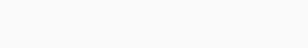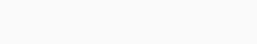The average queue length and the waiting time in the queue are given by: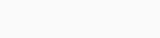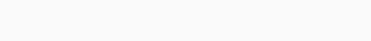## Exercise 4

A construction company has two identical machines, each of which can break down independently of the other following a rate Poisson process 5 times per month. It is assumed that the repair time is a rv which follows an exponential law with parameter mu (service rate). For what mu value, will the two machines be simultaneously in working order at least half the time?

The system is modeled by a birth and death process with 3 states (0, 1 or 2 broken down machines) with the following transition diagram: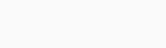We have :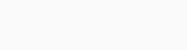From where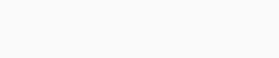p0 being the stationary probability so that the two machines are in working order, we must have:Taking into account the positivity of mu, we must have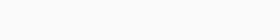It will therefore require a service rate at least equal to   13,660254 so that both machines are in working order at least every other time.

## Exercise 5

A laundry has 3 identical and independent machines. Each machine has an operating time independent of the past, following an exponential law of expectation of two days. A machine that breaks down is repaired by a technician; the duration of the repair is an expectation of one day. The laundry has only one technician.

Draw the transition diagram.

What is the fraction of time that all machines are running and that all machines are off?

What is the average number of machines in working order? What is the average number of immobilized machines?

1. Suppose that the state Ej designates the state in which j machines are immobilized. We have the following transition diagram: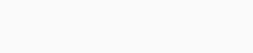2. We have in a steady state the following equations: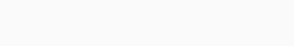From where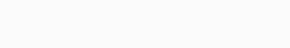The desired fractions are therefore 4/19 (for all the machines in operation) and 3/19 (for all the immobilized machines).

3. The average number of machines in working order is: 4 * (4/19) + 2 * (6/19) + 1 * (6/19) = 30/19. And the average number of immobilized machines is 9/19 + 12/19 + 6/193.

## Exercise 6

A database server receives an average of 100 requests per second, arriving in a Poisson process. The processing time of a request follows the exponential law of parameter µ. When the server is busy, requests are stored on a large disk for further processing on a “first come, first served” basis. There are 4 different types of servers on the market that can process 100, 150, 200 or 300 requests per second, respectively, when they are operating without interruption.

What will happen if you plan to install a server that can handle 100 requests per second?

We want a client who issues a request to have the response after 1/100 second on average. What type of server should I provide?

We agree that when there are already 8 requests stored in the queue, the new arriving requests would be too penalized in terms of response time. So we decide that in this case, they will be redirected instantly to an auxiliary server. To simplify, we will suppose that this new arrangement has a negligible influence on the old stationary distribution (calculated without auxiliary server). Calculate the probability that a request that arrives on the primary server will be redirected to the auxiliary server. How many, on average, will the auxiliary server receive requests per second?

This is an M / M / 1 queue, so we can use Little's laws. There is a stationary distribution if λ / μ <1, if we take a server which processes 100 / second then we have λ / μ = 1 the chain is not ergodic (infinite accumulation of requests)!

If we want a response time of 1/100 second then we have to solve the equation 1/100 = E (T) = 1 / (μ - λ) so μ = 200 requests / second.

Requests are redirected if there are already 8 requests in the system. So there is redirection when the system reaches 9 requests in the system. It suffices to calculate π9 to know the probability that the request is redirected. According to Little π's law9 = (1 - ρ) ρ9 =1/1024.

Since the system receives 100 requests per second, the auxiliary server receives 100/1024 requests per second.

## Exercise 7

In an automobile production plant, the problem of determining the optimum number of employees to be placed at the counters of a store responsible for supplying the necessary tools to the workers was studied. The store study began by determining the statistical characteristics of workers' arrivals (1.6 arrivals / minute) and the time spent by employees to provide the required tools (0.9 service / minute).

Calculate the average waiting time in the queue (Wq) for S = 2, S = 3, S = 4. Calculate the average number of customers in an 8-hour day and the corresponding average service time. Based on the number of employees (S), calculate the number of hours of inactivity per 8 hour day.

Calculate the time lost each day by workers, due to waiting? If the hourly cost of an employee is 40.00 \$ and that of a worker is 80.00 \$, what is the total cost of lost hours?

Conclude by giving the optimal number of employees to be expected for the store.

Knowing l and m, we have calculated l / m = 1.6 / 0.9 = 1.77> 1.

Since l / m> 1, we were only interested in the values of S = 2, S = 3, and S = 4.

To calculate the average waiting time in the queue, we first looked for P0, for each respective value of S.

S = 2, P0 = 0.061 S = 3, P0 = 0.152 S = 4, P0 = 0.166

Which give

S = 2, Wq = 4.00

S = 3, Wq = 0.31

S = 4, Wq = 0.06

Now let's calculate the average number of customers in an 8 hour day

wx 60 x 8 = 1.6 x 60 x 8 = 768

and, for this number of arrivals, it will be necessary (with a service time of 1 / m)

768 / m = 768 / 0.9 = 853 minutes of service per day.

= 14.21 hours

## Exercise 8

A laboratory contains 2 identical microcomputers. The users arrive according to a Poisson process with parameter l = 3 users per hour. The duration of use of a microcomputer is an exponential va of average 0.6 hour (m = 1 / 0.6).

- Laboratory utilization rate

- Number of users in the queue

- Waiting time in the queue

The boss finds that people waste too much time waiting, and decides to add microcomputers. He wants the average waiting time to be less than 20 minutes. How many should he add? What is the new laboratory utilization rate?

What would happen with regard to the waiting time of users if we separated them into 3 groups, assigning a microcomputer to each group?

What do you conclude?

We have an M / M / 2 queue.

r = l / 2m = 0.9

P0 = 1/19 P1 = l / m P0 = 1.8 / 19

Q = [r / 1-r] (1 - P0 - P1) = 7.674

Wq = 2.56 h

The system is only used at 90%, but each user has to wait on average more than 2 hours 30 minutes.

The boss finds that people waste too much time waiting and decides to add microcomputers. He wants the average waiting time to be less than 20 minutes. How many should he add?

We can try with several values of S = s, and calculate Wq for each case:

s = 3

Q = 0.532

Wq = 0.177 h. »10.6 min

With 3 microcomputers, the constraint is satisfied, but the system utilization rate is now r = 0.6.

What would happen if we separated the users into 3 groups, assigning a microcomputer to each group?

We now have 3 queues M / M / 1, each having an arrival rate l = 3/3 = 1, a service rate m = 1 / 0.6 and a utilization factor r = 0.6.

We obtain :

Wq = r² / [l (1-r)] = 0.9 hour

= 54 min.

It is therefore preferable to share the resources.

Thereby,

S = 2 employees would have 2 x 8 - 14.21 = 1.79 hours of inactivity per day

S = 3 employees would have 3 x 8 - 14.21 = 9.79 hours of inactivity per day

S = 4 employees would have 4 x 8 - 14.21 = 17.79 hours of inactivity per day

Let us now look for the time lost every day by the workers, due to the wait:

S = 2768 x 4 = 3072 min. = 51.2 hours

S = 3,768 x 0.31 = 238 min. = 3.96 hours

S = 4 768 x 0.06 = 46 min. = 0.76 hours

The total cost of the hours lost has the value:

S = 2 \$ 4 167.60 = 1.79 x 40 + 51.2 x 80

S = 3 \$ 708.40 = 9.79 x 40 + 3.96 x 80 the best one!

S = 4 \$ 772.40 = 17.79 x 40 + 0.76 X 80FRFRENES